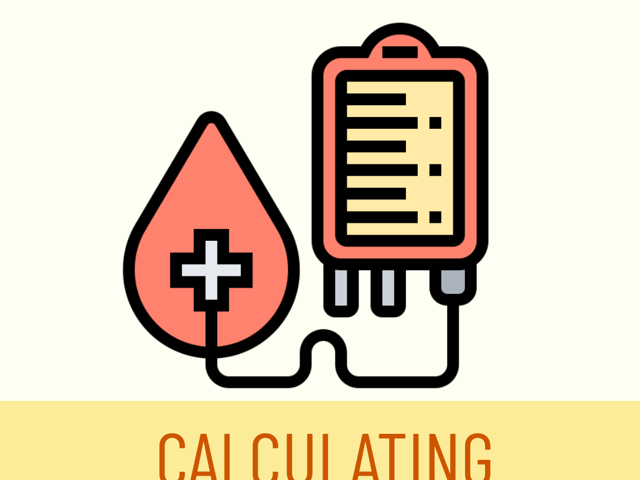# How to Calculate IV Drip Rates

In this day and age, electronic IV pumps have eliminated the need to manually calculate IV drip rates when administering fluids or medications. However, there may be a time when you do not have a functioning electronic pump and will need to make the conversions. And, because these fluids or medications are delivered directly to the patient’s bloodstream, there is little to no room for error.

## Information You’ll Need

When calculating an IV drip rate, the first step is to review the order. If the patient is going to receive fluids, the order will likely be to administer a certain amount of fluids over a specific amount of time (ex.: 250 mL/hr). When administering medications intravenously, the order will usually be in milligrams or micrograms per minute or hour, depending on the medication and dosage. And the medication will either be pre-mixed or need to be added to a bag of IV fluids. This will determine the concentration of the medication in either milligrams or micrograms per milliliter of fluid (ex.: 250mg/100mL).

## Tubing Selection

Next, you will select your IV tubing, which will depend on whether you are administering a higher volume of fluid or a lower volume of fluid. When administering a lower volume of fluid or needing to deliver a precise amount of the medication, you will use a micro-drip IV tubing set, which administers 60 drops (gtts) per mL. For higher volumes of fluid, you can use a macro-drip IV tubing set and will need to check the packaging, as they vary from 10-20 gtts/mL.

## Calculations

Using all of this information, you will perform the necessary conversions to calculate the number of drops per minute. As an example, you have an order to administer 150 mcg of Medication A over an hour, and your pharmacy has prepared Medication A in normal saline with a concentration of 750 mcg in 100 mL of normal saline and provided a microdrip tubing set.

First, let’s determine the liquid volume to be infused in an hour. To convert mcg/hr to mL/hr:

$\frac{150 \;\text{mcg}}{1 \;\text{hr}} \;\times \;\frac{100\; \text{mL}}{750 \;\text{mcg}}$

This equates to $$\frac{20 \;\text{mL}}{1 \;\text{hr}}$$.

Next, we will determine the drip rate. The formula is as follows:

$\text{Drip rate in gtt/minute} = \frac{\text{Total Milliliters}}{\text{Total Minutes}} \;\times \;\text{Drip factor in gtt/ml}$

So, in our example, you would do this:

$\frac{20 \;\text{mL}}{1 \;\text{hr}}\; \times \;\frac{1 \;\text{hr}}{60 \;\text{min}}\; \times\; \frac{60 \;\text{gtt}}{1\; \text{mL}}$

This equates to 20gtt/min or 20 drops per minute.

One interesting note: When using a micro-drip tubing set (60 drops per mL), the number of milliliters per hour will equal the number of drops per minute!